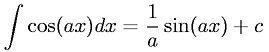Equations > Calculus > Integration/Integrals > Integral of cosine

### Integral of cosineLatex Code:

MathML Code:

 $\int \mathrm{cos}\left(\mathrm{ax}\right)\mathrm{dx}=\frac1a\mathrm{sin}\left(\mathrm{ax}\right)+c$

MathType 5.0: